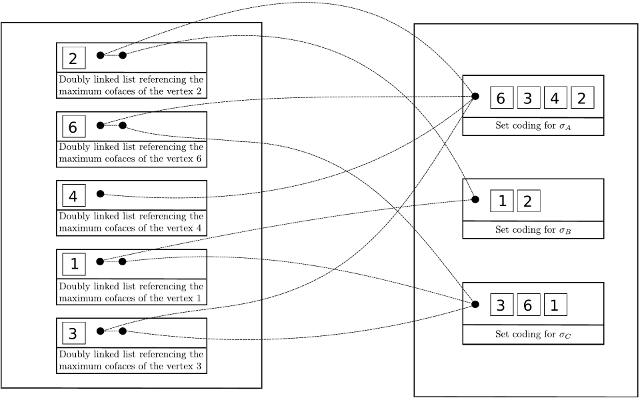Searching...
No Matches
Toplex Map

## Classes

class  Gudhi::Lazy_toplex_map
Lazy toplex map data structure for representing unfiltered simplicial complexes. More...

class  Gudhi::Toplex_map
Toplex map data structure for representing unfiltered simplicial complexes. More...

# Definition

A Toplex_map is a data structure to represent and store a simplicial complex. A "toplex" is the contraction of "top-simplex", also known as a maximal simplex. The plural form of "toplex" will be called "toplices".

Let's consider a simplicial complex, denote by $$d$$ its dimension and by $$k$$ its number of maximal simplices. Furthermore, denote by $$\Gamma_0$$ the maximal number of toplices, i.e. maximal simplices, that contain a same vertex.

The goal of the Toplex Map is both to represent the complex in optimal O(kd) space and to provide fast standard operations such as : insertion, removal, contraction of an edge, collapses and membership of a simplex. The time needed for these operation is linear or quadratic in $$\Gamma_0$$ and $$d$$.

Toplex map is composed firstly of a raw storage of toplices and secondly of a map which associate any vertex to a set of pointers toward all toplices containing this vertex. The data structure is described in  (aka. Simplex Array List or SAL).The performances are a lot better than the Simplex_tree as soon you use maximal simplices and not simplices (cf.  ).